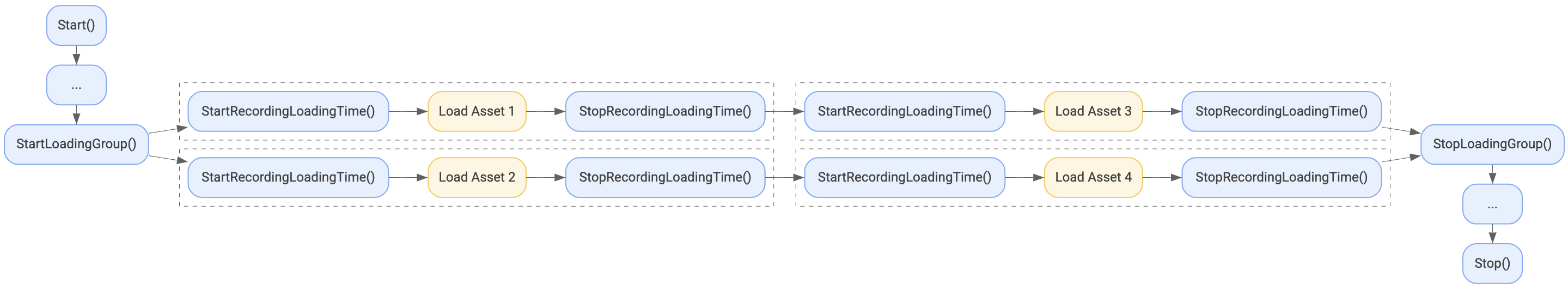2. To analyze load times to see when and where load times are longer than acceptable.

``````public class LoadingTimeMetadata
{
{
Unknown = 0,

/// <summary>
///     The first time the game is run.
/// </summary>
FirstRun = 1,

/// <summary>
///     App is not backgrounded.
/// </summary>
ColdStart = 2,

/// <summary>
///     App is backgrounded.
/// </summary>
WarmStart = 3,

/// <summary>
///     App is backgrounded, least work needed.
/// </summary>
HotStart = 4,

/// <summary>
/// </summary>
InterLevel = 5
}

{
UnknownSource = 0,

/// <summary>
///     Uncompressing data.
/// </summary>
Memory = 1,

/// <summary>
///     Reading assets from APK bundle.
/// </summary>
Apk = 2,

/// <summary>
///     Reading assets from device storage.
/// </summary>
DeviceStorage = 3,

/// <summary>
///     Reading assets from external storage, e.g. SD card.
/// </summary>
ExternalStorage = 4,

/// <summary>
/// </summary>
Network = 5,

/// <summary>
/// </summary>

/// <summary>
///     Time spent between process starting and onCreate.
/// </summary>
PreActivity = 7,

/// <summary>
///     Total time spent between process starting and first render frame.
/// </summary>
FirstTouchToFirstFrame = 8,

/// <summary>
///     Time from start to end of a group of events.
/// </summary>
TotalUserWaitForGroup = 9
}

/// <summary>
///     0 = no compression, 100 = max compression
/// </summary>
public int compression_level;

public enum NetworkConnectivity
{
Unknown = 0,
Wifi = 1,
CellularNetwork = 2
}

public NetworkConnectivity network_connectivity;

/// <summary>
///     Bandwidth in bits per second.
/// </summary>
public ulong network_transfer_speed_bps;

/// <summary>
///     Latency in nanoseconds.
/// </summary>
public ulong network_latency_ns;
}
``````

Any fields that aren't relevant to your needs can be zero.

A loading event can also have an associated annotation. You can define in the same way as frame time annotations, using one or more fields in the `Annotation` message.

```Result<ulong> StartRecordingLoadingTime(LoadingTimeMetadata eventMetadata, TAnnotation annotation);```

This function starts recording a loading time event associated with the given metadata and annotation, and fills in a `Result<ulong>.value` to be used in the `StopRecordingLoadingTime()` function.

`ErrorCode StopRecordingLoadingTime(ulong handle);`

This function stops recording an event previously started by `StartRecordingLoadingTime()`. The event is uploaded at the next session flush.

It is important to inform Android Performance Tuner that loading events are part of such a group so that it can provide better insights. Bracket your loading events with the following start and stop functions in order to do this.

```Result<ulong> StartLoadingGroup(LoadingTimeMetadata eventMetadata, TAnnotation annotation);```

This function starts a loading group associated with the given metadata and annotation, and fills in a `Result<ulong>.value` to be used in the `StopLoadingGroup()` function. The metadata and annotation are currently not used by the Play backend but only the annotation can be set to `null`. All subsequent loading events are tagged by a unique group ID.

`ErrorCode StopLoadingGroup(ulong handle);`

This function stops a loading group previously started by `StartLoadingGroup()`. Subsequent loading events will not have a group ID until `StartLoadingGroup()` is called again.Figure 1. Example of the loading group.

## Examples

``````public RawImage image;

{
string imagePath = "file://" + Path.Combine(Application.streamingAssetsPath, imageName);
using (var r = UnityWebRequestTexture.GetTexture(imagePath))
{
{
// Fields are zero by default but they could be set as follows
compression_level = 0,
network_connectivity = 0,
network_transfer_speed_bps = 0,
network_latency_ns = 0
};
Annotation annotation = new Annotation()
{
Scene = Scene.MagicalForest
};
yield return r.SendWebRequest();
if (r.isNetworkError || r.isHttpError)
{
Debug.Log(r.error);
}
else
{
image.texture = tex;
}
}
}
``````

``````IEnumerator LoadScene(int sceneIndex)
{
Annotation annotation = new Annotation() {Scene = (Scene) (sceneIndex + 1)};
{
yield return null;
}

}
``````

``````IEnumerator LoadImages()
{
{